Two positively charged particles are separated on an axis by 0.1 meters, and q1 has a 6 µC charge and q2 has a 2 µC charge. Recall that k =

Question

Two positively charged particles are separated on an axis by 0.1 meters, and q1 has a 6 µC charge and q2 has a 2 µC charge. Recall that k = 8.99 × 109 N • meters squared over Coulombs squared.. What is the force applied between q1 and q2 in N? In which direction does particle q2 want to go?

in progress 0
5 months 2021-08-19T05:08:34+00:00 2 Answers 30 views 0

10.8 N & away from particle q1, in a straight line to the right

Explanation:

•°•°•°

Force between two charges is 10.8 N

both charges are positive nature charge so they both repel each other and hence q2 will move away from charge q1

Explanation:

As we know that the force between two charge particles is given by Coulomb’s law of electrostatics

So we will say it as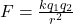here we know that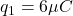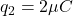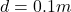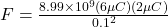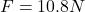Since both charges are positive nature charge so they both repel each other and hence q2 will move away from charge q1